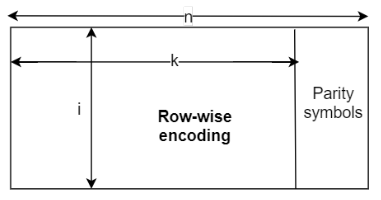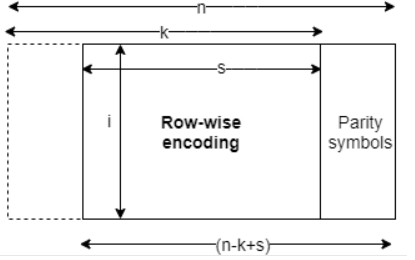# ccsdsRSDecode

Decode CCSDS-complaint RS codes

Since R2021a

## Syntax

``[decoded,cnumerr,ccode] = ccsdsRSDecode(code,k)``
``[decoded,cnumerr,ccode] = ccsdsRSDecode(code,k,i)``
``[decoded,cnumerr,ccode] = ccsdsRSDecode(code,k,i,s)``

## Description

example

````[decoded,cnumerr,ccode] = ccsdsRSDecode(code,k)` decode the received signal in `code` by using a (255, `k`) Reed-Solomon (RS) decoder with the generator polynomial, as defined in the Consultative Committee for Space Data Systems (CCSDS) 131.0-B-3 Section 4 . `k` is the number of symbols in the decoded message. The function returns the decoded message `code`, `decoded`, the number of corrected errors, `cnumerr`, and the corrected version of `code`, `ccode`. For a description of CCSDS RS code decoding, see CCSDS RS Code Decoding.```

example

````[decoded,cnumerr,ccode] = ccsdsRSDecode(code,k,i)` specifies the interleaving depth, `i`. `code` consists of `i` RS codewords of length 255 bytes.```
````[decoded,cnumerr,ccode] = ccsdsRSDecode(code,k,i,s)` specifies the shortened message length, `s`.```

## Examples

collapse all

Generate a full-length encoded Reed-Solomon (RS) codeword, introduce random errors, and decode the result using a Consultative Committee for Space Data Systems (CCSDS) RS decoder.

Generate a random message of length `k`.

```k = 223; msg = randi([0 255],k,1);```

Encode the message by using a CCSDS RS encoder.

`code = ccsdsRSEncode(msg,k);`

Generate 15 random error symbols and 15 unique random locations to insert these errors.

```err = randi([1 255],15,1); errLoc = randperm(255,15); errVec = zeros(255,1); errVec(errLoc) = err;```

Introduce error symbols in the encoded message.

`rxBytes = bitxor(code,errVec);`

Decode the encoded symbols introduced with errors by using CCSDS RS decoder.

`[decoded,v,ccode] = ccsdsRSDecode(rxBytes, k);`

Display the number of corrected errors.

`disp(v)`
``` 15 ```

Generate an full-length encoded Reed-Solomon (RS) codeword, introduce burst of erros, and decode the result using a Consultative Committee for Space Data Systems (CCSDS) RS decoder.

Specify the message length `k` and interleaving depth, `i`.

```k = 239; i = 5;```

Generate a column vector of random message bits. Encode the shortened message by using a CCSDS RS encoder.

```msg = randi([0 255],k*i,1); code = ccsdsRSEncode(msg,k,i);```

Generate 30 random error symbols.

```err = randi([1 255],30,1); errVec = zeros(255*i,1);```

Introduce burst errors from location 52 to 81.

```errVec(52:81) = err; rxBytes = bitxor(code,errVec);```

Decode the encoded symbols introduced with burst errors by using a CCSDS RS decoder.

`[decoded,v,ccode] = ccsdsRSDecode(rxBytes,k,i);`

Display the number of corrected errors.

`disp(v)`
``` 30 ```

## Input Arguments

collapse all

Encoded message, specified as a column vector of integers in the range [0, 255].

The elements and the size of the column vector depends on the data type of the input message.

• For a logical data type, each element in the vector is either 0 or 1.

• For a uint8 or double data type, each element is an integer symbol in GF(2m), in the range [0, 255]. m is the number of bits in each symbol.

Input Message TypeSize of `code`
Data Type of `code` Is logicalData Type of `code` Is uint8 or double
Full length input message8*255 255
Interleaved input message8*255*`i` 255*`i`
Shortened input message8*`i`*(255 – `k` + `s`)`i`*(255 – `k` + `s`)

Data Types: `double` | `uint8` | `logical`

Number of symbols in the decoded message, specified as `223` or `239`.

Data Types: `double`

Interleaving depth, specified as `1`, `2`, `3`, `4`, `5`, or `8`. The default value, `1`, corresponds to no interleaving.

`code` consists of `i` RS codewords of length 255 bytes.

Data Types: `double`

Shortened message length, specified as an integer in the range [1, `k`].

Data Types: `double`

## Output Arguments

collapse all

Decoded message, returned as a column vector. Each element represents decoding the corresponding element in input `code`. The data type of `decoded` is the same as that of `code`.

The size of the column vector depends on the data type of `code`.

Input Message TypeSize of `decoded`
Data Type of `code` Is logicalData Type of `code` Is uint8 or double
Full length input message8*`k` `k`
Interleaved input message8*`k`*`i` `k`*`i`
Shortened input message8*`s`*`i` `s`*`i`

When the value of output `cnumerr` is `–1`, `decoded` is equal to the first `k` elements of `code`.

Number of corrected errors, returned as an integer in the range [`-`1, (n`k`) ∕ 2], where n is the codeword length. The value of n is set to `255` according to CCSDS 131.0-B-3 Section 4 .

A value of `–1` in `cnumerr` indicates the failure of the decoder to correct the errors.

Corrected version of code, returned as a column vector. The length of `ccode` is same as the length of `code`. The data type of `ccode` is the same as that of `code`.

When the value of output `cnumerr` is `–1`, `ccode` is equal to `code`.

collapse all

### CCSDS RS Code Decoding

CCSDS RS codes are powerful burst error-correcting codes. These are most commonly used as forward error-correcting (FEC) codes, as they detects and correct errors on the symbol level.

Decoding Full-Length Message CCSDS RS Codes

Like encoding, decoding of CCSDS RS codes is also done row-wise. The input vector length is a product of interleaving depth (i) and codeword length (n). n is fixed to 255 symbols according to CCSDS 131.0-B-3 Section 4 . The input vector is composed of message and parity symbols.Decoding Shortened Message CCSDS RS Codes

Like encoding, the decoding of CCSDS RS codes is also done row-wise. The input vector length is a product of the interleaving depth (i) and the value calculated by n-k+s. The input vector is composed of shortened message and parity symbols.TM Synchronization and Channel Coding. Recommendation for Space Data System Standards. CCSDS 131.0-B-3. Blue Book. Issue 3. Washington, D.C.: CCSDS, September 2017.